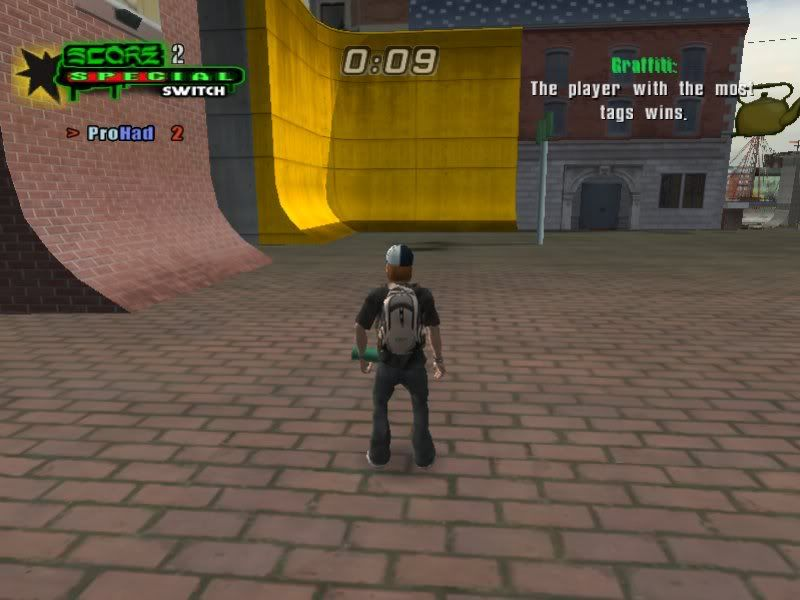## [THUG+][PC]Changing Graffiti Colours

Tutorials for all THUG+ games goes here.
Forum rules
This forum is only for posting tutorials.
Specify what game it is for, and please take a look at this Template.
VIP
Posts: 45
Joined: Wed Mar 03, 2010 10:10 pm

### [THUG+][PC]Changing Graffiti Colours

What you need:
qb2.exe
gameoptions.qb

Decompile gameoptions.qb useing

qb2.exe -d gameoptions.qb>gameoptions.txt
When you open up gameoptions.txt it will be the very first thing you see.

:i \$graffitiColors\$ = :a{
:i :a{%i(128,00000080)%i(128,00000080)%i(128,00000080)%i(100,00000064):a}
:i :a{%i(102,00000066)%i(26,0000001a)%i(8,00000008)%i(128,00000080):a}
:i :a{%i(17,00000011)%i(64,00000040)%i(116,00000074)%i(128,00000080):a}
:i :a{%i(9,00000009)%i(107,0000006b)%i(36,00000024)%i(128,00000080):a}
:i :a{%i(123,0000007b)%i(99,00000063)%i(3,00000003)%i(128,00000080):a}
:i :a{%i(0,00000000)%i(128,00000080)%i(128,00000080)%i(128,00000080):a}
:i :a{%i(128,00000080)%i(0,00000000)%i(128,00000080)%i(128,00000080):a}
:i :a{%i(80,00000050)%i(80,00000050)%i(116,00000074)%i(128,00000080):a}
:i :a{%i(88,00000058)%i(105,00000069)%i(112,00000070)%i(128,00000080):a}
:i :a}

:i \$graffitiColors\$ = :a{
:i :a{%i(128,00000080)%i(128,00000080)%i(128,00000080)%i(100,00000064):a}
:i :a{%i(102,00000066)%i(26,0000001a)%i(8,00000008)%i(128,00000080):a}
:i :a{%i(17,00000011)%i(64,00000040)%i(116,00000074)%i(128,00000080):a}
:i :a{%i(9,00000009)%i(107,0000006b)%i(36,00000024)%i(128,00000080):a}
:i :a{%i(123,0000007b)%i(99,00000063)%i(3,00000003)%i(128,00000080):a}
:i :a{%i(0,00000000)%i(128,00000080)%i(128,00000080)%i(128,00000080):a}
:i :a{%i(128,00000080)%i(0,00000000)%i(128,00000080)%i(128,00000080):a}
:i :a{%i(80,00000050)%i(80,00000050)%i(116,00000074)%i(128,00000080):a}
:i :a{%i(88,00000058)%i(105,00000069)%i(112,00000070)%i(128,00000080):a}
:i :a}
now everything except for the top line i highlighted are the players graf colours, to be honest with you i have no idea what the top line is, but apparently it's not important at all because i've already done this.

so if we wanted to make first players graf colour a nice orange we'd take the following line.

:a{%i(102,00000066)%i(26,0000001a)%i(8,00000008)%i(128,00000080):a}
and find a good looking orange on this site: http://www.farb-tabelle.de/de/farbtabelle.htm

i picked 255,165,0

the charts from that site are set up RGB

so it would be R:255 G:165 B:0

The THPS scripts are set up the same way

:a{%i(102,00000066)%i(26,0000001a)%i(8,00000008)%i(128,00000080):a}

Red, Green, Blue, Alpha

So the red for the orange i chose would be 225 so we change the 102 to 225 convert that to hex it's e1 so we replace the 66 with e1, do the same with green and blue(i suggest you just leave the alpha) and you'll have orange graf colour.

your line would end up looking like this.

:a{%i(225,000000e1)%i(165,000000a5)%i(0,00000000)%i(128,00000080):a}dennis344
Posts: 18
Joined: Thu Jun 17, 2010 10:42 pm

### Re: Changing Graffiti Colours

I did exactly what you did, put the gameoptions in my folder and nothing changed.
Any reason why?